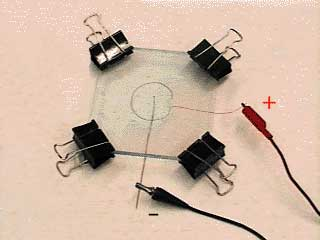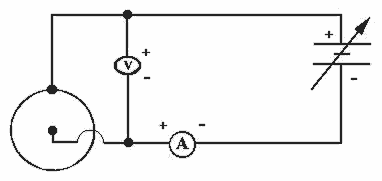HandsOn 13 - Growing a Pattern in the Laboratory

I. Introduction

I. Introduction

In this experiment, supplied in the accompanying laboratory kit, you have the opportunity to grow a physical object and measure its fractal dimension by two independent methods. The electrochemical deposition experiment is abbreviated ECD. The ECD experiment is carried out in an ECD cell consisting of two parallel plastic plates (Figure 4.1). Between these plates a circular positive terminal surrounds a central negative terminal. The space between the plates is the thickness of the positive terminal wire, about 1/2 mm or 500 micrometers. Between the plates, and between the electrodes, is a salt solution. Most likely you will be using a solution of copper sulfate (Cu2SO4) or zinc sulfate (Zn2SO4). This apparatus is an electrolytic cell, one that requires a current input (as opposed to an electrochemical cell such as a battery-a galvanic cell-which spontaneously produces a current). The circuit is shown in Figure 4.2.

 Q4.4: Speculate: What will happen at the negative terminal? If you expect something to grow, sketch what you expect it to look like as it grows. Draw three stages of its development in your laboratory notebook: early, halfway through, and completed. Explain why you expect it to appear the way you have drawn it.Figure 4.1: The electrodeposition cell. The circular loop of wire is the positive terminal, surrounding the central negative terminal. The space between the positive terminal and the negative terminal is filled with an electrolytic solution. The spacing between the plates is the same as the thickness of the positive terminal wire loop. The negative terminal wire extends vertically through both plates.Figure 4.2: Circuit diagram for electrodeposition experiment. The electrodeposition cell is shown in the lower left-hand corner. The meters labeled A and V measure the current and voltage, respectively. An adjustable voltage power supply is represented by the battery symbol with an arrow across it.

 Q4.5: Hypothesize: What carries the current in the wires of the circuit? What carries the current through the cell? When the cell is hooked up, will current flow as long as the power supply stays turned on? Does the electrolytic solution develop a net charge?

 Q4.6: How can you determine the number of metal atoms being deposited per second at the negative terminal? Data needed to answer this question can be obtained with the meters shown in the circuit of Figure 4.2.

 Q4.7: Without using a balance, how can you determine experimentally the mass of the atoms deposited per second at the negative terminal? Is there a way to use the current meter to do this? Do you expect the mass deposited per second to vary with time?

If you are working in a classroom setting, you will need at least one partner, and preferably two partners, for this experiment. In addition to the equipment provided in the kit, you will need a power source with variable voltage (e.g., between 1 and 20 Volts) and current (between 10 and 250 milliamps).

Previous: 4.1 - Electrodeposition

Next: II. Setting Up the Experiment